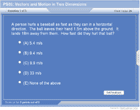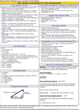How to Learn in 24 Hours?The Rapid Learning Movie

 Need Help? M-F: 9am-5pm(PST): Toll-Free: (877) RAPID-10 US Direct: (714) 692-2900 Int'l: 001-714-692-2900 24/7 Online Technical Support: The Rapid Support Center Secure Online Order:Need Proof? Testimonials by Our Users

 Rapid Learning Courses: MCAT in 24 Hours (2015-16) USMLE in 24 Hours (Boards) Chemistry in 24 Hours Biology in 24 Hours Physics in 24 Hours Mathematics in 24 Hours Psychology in 24 Hours SAT in 24 Hours ACT in 24 Hours AP in 24 Hours CLEP in 24 Hours DAT in 24 Hours (Dental) OAT in 24 Hours (Optometry) PCAT in 24 Hours (Pharmacy) Nursing Entrance Exams Certification in 24 Hours eBook - Survival Kits Audiobooks (MP3)

 Tell-A-Friend: Have friends taking science and math courses too? Tell them about our rapid learning system.Home »  Physics »  High School Physics

Vectors and Motion in Two Dimensions

 Topic Review on "Title": Vector:  A quantity that has magnitude, size, and direction. Velocity and displacement are examples. Scalar:  A quantity that has only magnitude, or size.  Speed, distance, and time are examples. Vector Addition: When vectors are graphically added, they are drawn head to tail.  This may also be described as placing the arrowhead of one vector next to the tail end of another vector. Resultant: The result of adding two or more vectors; vector sum.  It is drawn from the tail of the first vector to the tip of the last vector. Phythagorean Theorem: c2=a2+b2 Vector Component: The parts into which a vector can be separated and that act in different directions from the vector. Horizontal Motion of a Projectile: Since gravity acts only vertically, there is 0 acceleration. Horizontally, the projectile moves with a constant speed. We can use one of the previous kinematic formulas  v=d/t Vertical Motion of a Projectile: Use previous kinematic formulas in the vertical direction. a = Δv/Δt=(vf-vi)/t d=vit+at2/2 vf2=vi2+2ad acceleration due to gravity = -9.8 m/s2

Rapid Study Kit for "Title":
 Flash Movie Flash Game Flash Card Core Concept Tutorial Problem Solving Drill Review Cheat Sheet"Title" Tutorial Summary : This tutorial describes motion in two dimensions.  The concepts of vector and scalar will be emphasized, espically vector addition and vector components.  These ideas will be applied to various projectile or motion in 2 dimension problems.  In these examples you will see how gravity only affects the vertical motion of a projectile.  Any horizontal motion of a projectile will remain unchanged as long as air resistance is neglected.  You will also see how to apply revious kinematic formulas to these new 2 dimensional situations.

 Tutorial Features: Specific Tutorial Features: • Graphically see vectors added or broken into components. • Problem-solving techniques are used to work out the example problems. Series Features: • Concept map showing inter-connections of new concepts in this tutorial and those previously introduced. • Definition slides introduce terms as they are needed. • Visual representation of concepts • Animated examples—worked out step by step • A concise summary is given at the conclusion of the tutorial.

 "Title" Topic List: Vectors Definiton and notation Addition of vectors Components Projectile Motion Horizontally fired projectile, detailed examples Angled projectile, detailed examples

See all 24 lessons in high school  physics, including concept tutorials, problem drills and cheat sheets:
Teach Yourself High School Physics Visually in 24 Hours